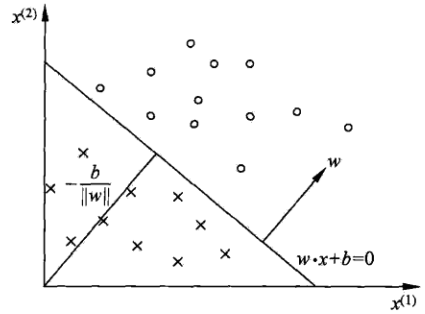# 简介

1957年Rosenblatt提出感知机（perceptron），成为神经网络和支持向量机的基础。感知机是二类分类的线性分类模型，输入是实例的特征向量，输出是实例的类别。感知机学习的目标是求出将训练数据进行线性划分的分离超平面，感知机属于判别模型。

# 感知机模型# 感知机学习算法

## 感知机学习算法的原始形式

1. $\nabla _w L(w,b) = -\sum_x y_i x_i$
2. $\nabla_b L(w,b) = -\sum_x y_i$

1. $w \leftarrow w + \eta y_i x_i$
2. $b\leftarrow \eta y_i$
其中，$\eta(0<\eta \ge 1)$是步长，又称为学习率。这样，通过迭代以期损失函数$L(w,b)$不断减小，直到0为止。

1. 选取初值
2. 在训练集中选取数据
3. 如果 $y_i(w \cdot x_i + b) \le 0$: $w \leftarrow w + \eta y_i x_i$;$b\leftarrow \eta y_i$
4. 转至2，直至训练集中没有误分类点。

1. 好的
2. 电话